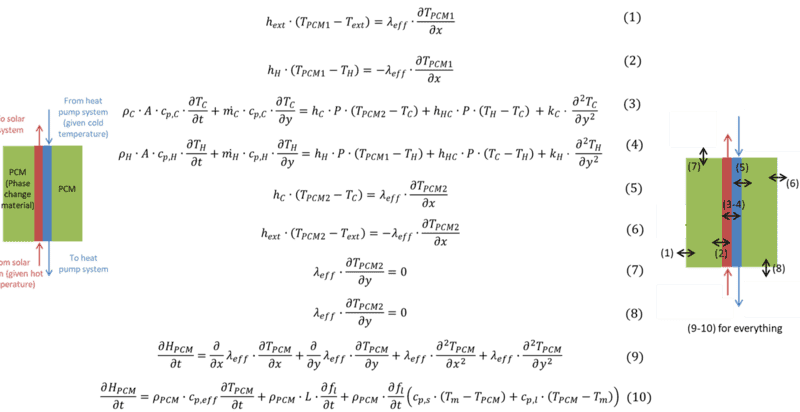# Heat exchange in a thermal storage based on phase change materials

• A
• DianeLR

#### DianeLR

Hello,

I want to model a thermal battery based on phase change materials (PCM). It is a plate heat exchanger immersed in a PCM bath. The diagram is given in the attached file.
I want to determine the temperature at each moment and from everywhere in the battery. The hypotheses are the following:

- Natural convection neglected (pure conduction),
- No supercooling or superheating,
- incompressible and Newtonian heat transfer fluid,
- Kinetic and potential energy variations are neglected,
- Homogeneous, isotropic and pure body PCM,
- Isothermal phase change,
- Density variation of PCM during the change of state neglected,
- Thermophysical properties independent of temperature (and different for liquid and solid phases).

These assumptions allowed me to obtain the equations visible in the attached file. The MCP parts are modeled in 2D (in x and y) while the heat transfer fluids in 1D (in y).
Do the equations seem correct to you?#### Attachments

I kind of see what you are solving here, but part of it confuses me.

The are two regions of PCM, 1 and 2. Region 1 is next to the hot side of the heat exchanger and 2 is next to the cold side. Both regions can have solid (at temperatures below the melting point), liquid at temperatures above the melting point, or a combination of solid and liquid (liquid fraction f) at the melting point. Correct so far?

I have a problem with Eqn. 10. The enthalpy per unit mass behavior should be as follows:

##h=c_s(T-T_{M})## for ##T<T_M##

##h=Lf## for ##T=T_M##

##h=L+c_l(T-T_M)## for ##T>T_M##

Are you assuming that solid and liquid can exist together at temperatures other than the melting point?

In Eqns. 3 and 4, the final terms on the RHS (axial conduction terms) are negligible except for liquid metals.

It's correct what you said.
For the enthalpie, I used that equation to determine the equation inside the PCM (see picture)

And thanks for the equations (3) and (4). It will be easier to solve with one less term

#### Attachments

I think I am making a mistake with equation (4). The hot heat transfer fluid is against the flow of the cold. Shouldn't there be a minus sign before $$m c_p$$ (on the left of the equality)?

For the enthalpie, I used that equation to determine the equation inside the PCM (see picture)
It doesn't look the same as what I gave.

It might be easier to integrate 9 and 10 in terms of enthalpy than in terms of temperature.
I think I am making a mistake with equation (4). The hot heat transfer fluid is against the flow of the cold. Shouldn't there be a minus sign before $$m c_p$$ (on the left of the equality)?
Yes

Thank you for your response. I will check for the integration in terms of enthalpy.